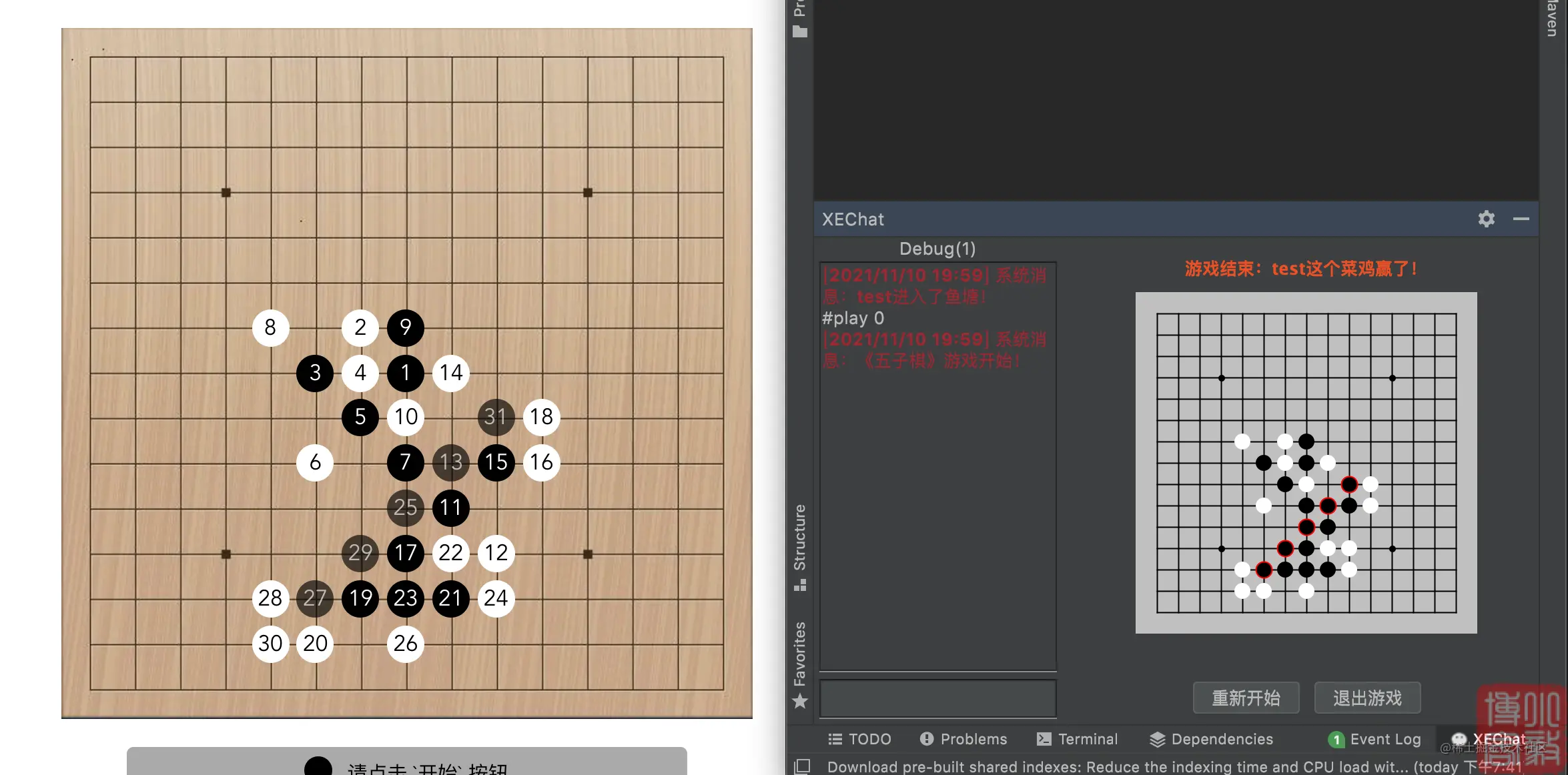# 教你写一个入门级别的五子棋AI

## 前言## 实现过程

### 抽象

``````/**
* 棋子点位
*
* @author anlingyi
* @date 2021/11/10
*/@AllArgsConstructor@NoArgsConstructor@ToString
public class Point {/**
* 横坐标
*/int x;/**
* 纵坐标
*/int y;/**
* 棋子类型 1.黑 2.白
*/int type;
}``````

`AI` 对外提供的接口，不会依赖任何 `GUI` 代码，方便其他程序调用。

``````/**
* 五子棋AI接口
*
* @author anlingyi
* @date 2021/11/10
*/public interface AIService {/**
* 获取AI棋位
*
* @param chessData 已下棋子数据
* @param point     对手棋位
* @param started   是否刚开局
* @return
*/Point getPoint(int[][] chessData, Point point, boolean started);
}``````

### 实现AI接口

``````/**
*
* 五子棋AI实现
*
* @author anlingyi
* @date 2021/11/10
*/public class ZhiZhangAIService implements AIService {/**
* 已下棋子数据
*/private int[][] chessData;/**
* 棋盘行数
*/private int rows;/**
* 棋盘列数
*/private int cols;/**
* AI棋子类型
*/private int ai;/**
* 声明一个最大值
*/private static final int INFINITY = 999999999;@Overridepublic Point getPoint(int[][] chessData, Point point, boolean started) {// 初始化棋盘数据
initChessData(chessData);// 计算AI的棋子类型this.ai = 3 - point.type;if (started) {// AI先下，首子天元int centerX = this.cols / 2;int centerY = this.rows / 2;return new Point(centerX, centerY, this.ai);
}// 获取最佳下棋点位return getBestPoint();
}/**
* 初始化棋盘数据
*
* @param chessData 当前棋盘数据
*/private void initChessData(int[][] chessData) {// 获取棋盘行数this.rows = chessData.length;// 获取棋盘列数this.cols = chessData.length;// 初始化棋盘数据this.chessData = new int[this.cols][this.rows];// 深拷贝for (int i = 0; i < cols; i++) {for (int j = 0; j < rows; j++) {this.chessData[i][j] = chessData[i][j];
}
}
}/**
* 获取最佳下棋点位
*
* @return
*/private Point getBestPoint() {
Point best = null;// 初始分值为最小int score = -INFINITY;/* 遍历所有能下棋的点位，评估各个点位的分值，选择分值最大的点位 */for (int i = 0; i < this.cols; i++) {for (int j = 0; j < this.rows; j++) {if (this.chessData[i][j] != 0) {// 该点已有棋子，跳过continue;
}
Point p = new Point(i, j, this.ai);// 评估该点AI得分int val = evaluate(p);// 选择得分最高的点位if (val > score) {// 最高分被刷新
score = val;// 更新最佳点位
best = p;
}
}
}return best;
}/**
* 对当前棋位进行评估
*
* @param point 当前棋位
* @return
*/private int evaluate(Point point) {// 核心
}
}``````

``this.ai = 3 - point.type;``

``````if (started) {// AI先下，首子天元
int centerX = this.cols / 2;
int centerY = this.rows / 2;return new Point(centerX, centerY, this.ai);
}``````

``````    private Point getBestPoint() {
Point best = null;// 初始分值为最小
int score = -INFINITY;/* 遍历所有能下棋的点位，评估各个点位的分值，选择分值最大的点位 */for (int i = 0; i < this.cols; i++) {for (int j = 0; j < this.rows; j++) {if (this.chessData[i][j] != 0) {// 该点已有棋子，跳过continue;
}
Point p = new Point(i, j, this.ai);// 评估该点AI得分
int val = evaluate(p);// 选择得分最高的点位if (val > score) {// 最高分被刷新
score = val;// 更新最佳点位
best = p;
}
}
}return best;
}``````

``````   /**
* 对当前棋位进行评估
*
* @param point 当前棋位
* @return
*/private int evaluate(Point point) {// 核心
}``````

### 评估函数

``0:空位 1:黑子 2:白子连五：11111活四：011110冲四：21111活三：001110眠三：211100活二：001100眠二：001120眠一：001200``

`冲四``活三` 如果形成，赢的可能性很大，`活四` 如果形成，棋局胜负基本确定，`连五` 形成就已经赢了。所以说，如果 `AI` 落的点能够形成这几种胜率很高的棋型的话，我们要给这个点评一个高分，这样对 `AI` 最有利。

#### 评估模型的抽象

``````    @AllArgsConstructorprivate enum ChessModel {/**
* 连五
*/
LIANWU(10000000, new String[]{"11111"}),/**
* 活四
*/
HUOSI(1000000, new String[]{"011110"}),/**
* 活三
*/
HUOSAN(10000, new String[]{"001110", "011100", "010110", "011010"}),/**
* 冲四
*/
CHONGSI(8000, new String[]{"11110", "01111", "10111", "11011", "11101"}),/**
* 眠三
*/
MIANSAN(1000, new String[]{"001112", "010112", "011012", "211100", "211010"}),/**
* 活二
*/
HUOER(800, new String[]{"001100", "011000", "000110"}),/**
* 眠二
*/
MIANER(50, new String[]{"011200", "001120", "002110", "021100", "001010", "010100"}),/**
* 眠一
*/
MIANYI(10, new String[]{"001200", "002100", "020100", "000210", "000120"});/**
* 分数
*/
int score;/**
* 局势数组
*/String[] values;
}``````

``````    /**
* 棋型分数表
*/private static final Map SCORE = new LinkedHashMap<>();static {// 初始化棋型分数表for (ChessModel chessScore : ChessModel.values()) {for (String value : chessScore.values) {
SCORE.put(value, chessScore.score);
}
}
},>``````

#### 判断落子点位的棋型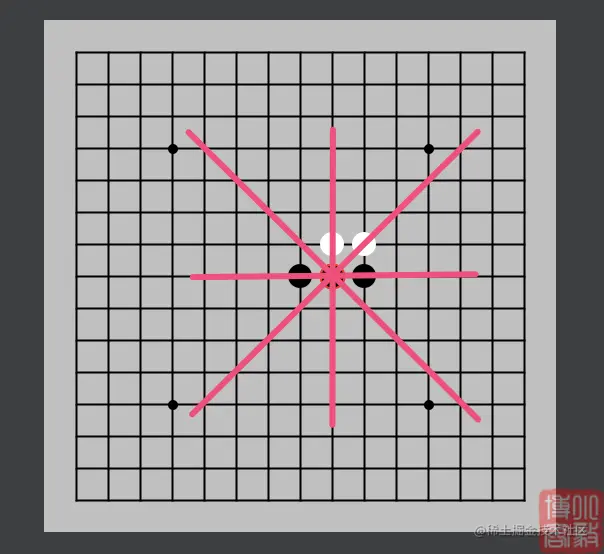``````横：000111000 -> 活三 +10000

``10000 + 10 + 10 + 0 = 10020``

``````    /**
* 获取局势分数
*
* @param situation 局势
* @return
*/private int getScore(String situation) {for (String key : SCORE.keySet()) {if (situation.contains(key)) {return SCORE.get(key);
}
}return 0;
}/**
* 获取棋位局势
*
* @param point     当前棋位
* @param direction 大方向 1.横 2.纵 3.左斜 4.右斜
* @return
*/private String getSituation(Point point, int direction) {// 下面用到了relativePoint函数，根据传入的四个大方向做转换
direction = direction * 2 - 1;// 以下是将各个方向的棋子拼接成字符串返回
StringBuilder sb = new StringBuilder();
appendChess(sb, point, direction, 4);
appendChess(sb, point, direction, 3);
appendChess(sb, point, direction, 2);
appendChess(sb, point, direction, 1);
sb.append(1); // 当前棋子统一标记为1(黑)
appendChess(sb, point, direction + 1, 1);
appendChess(sb, point, direction + 1, 2);
appendChess(sb, point, direction + 1, 3);
appendChess(sb, point, direction + 1, 4);return sb.toString();
}/**
* 拼接各个方向的棋子
* ``````

``` * 由于现有评估模型是对黑棋进行评估 * 所以，为了方便对局势进行评估，如果当前是白棋方，需要将扫描到的白棋转换为黑棋，黑棋转换为白棋 * 如：point(x=0,y=0,type=2) 即当前为白棋方 * 扫描到的某个方向局势为：20212 -> 转换后 -> 10121 * * @param sb 字符串容器 * @param point 当前棋子 * @param direction 方向 1.左横 2.右横 3.上纵 4.下纵 5.左斜上 6.左斜下 7.右斜上 8.右斜下 * @param offset 偏移量 */private void appendChess(StringBuilder sb, Point point, int direction, int offset) {int chess = relativePoint(point, direction, offset);if (chess > -1) {if (point.type == 2) {// 对白棋进行转换if (chess > 0) {// 对棋子颜色进行转换，2->1，1->2 chess = 3 - chess; } } sb.append(chess); } }/** * 获取相对点位棋子 * * @param point 当前棋位 * @param direction 方向 1.左横 2.右横 3.上纵 4.下纵 5.左斜上 6.左斜下 7.右斜上 8.右斜下 * @param offset 偏移量 * @return -1:越界 0:空位 1:黑棋 2:白棋 */private int relativePoint(Point point, int direction, int offset) {int x = point.x, y = point.y;switch (direction) {case 1: x -= offset;break;case 2: x += offset;break;case 3: y -= offset;break;case 4: y += offset;break;case 5: x += offset; y -= offset;break;case 6: x -= offset; y += offset;break;case 7: x -= offset; y -= offset;break;case 8: x += offset; y += offset;break; }if (x < 0 || y < 0 || x >= this.cols || y >= this.rows) {// 越界return -1; }// 返回该位置的棋子return this.chessData[x][y]; }```

#### 评估函数的实现

``````    /**
* 对当前棋位进行评估
*
* @param point 当前棋位
* @return
*/private int evaluate(Point point) {// 分值int score = 0;for (int i = 1; i < 5; i++) {// 获取该方向的局势
String situation = getSituation(point, i);// 下此步的得分
score += getScore(situation);
}return score;
}``````

``````    /**
* 获取AI棋位
*
* @param chessData 已下棋子数据
* @param point     对手棋位
* @param started   是否刚开局
* @return
*/Point getPoint(int[][] chessData, Point point, boolean started);``````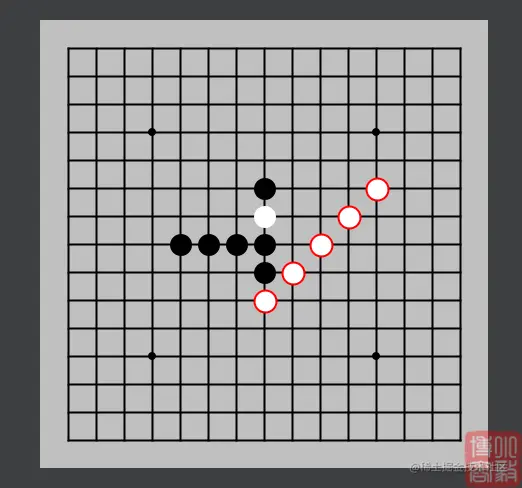``````    private Point getBestPoint() {
Point best = null;// 初始分值为最小
int score = -INFINITY;/* 遍历所有能下棋的点位，评估各个点位的分值，选择分值最大的点位 */for (int i = 0; i < this.cols; i++) {for (int j = 0; j < this.rows; j++) {if (this.chessData[i][j] != 0) {// 该点已有棋子，跳过continue;
}
Point p = new Point(i, j, this.ai);// 该点得分 = AI落子得分 + 对手落子得分
int val = evaluate(p) + evaluate(new Point(i, j, 3 - this.ai));// 选择得分最高的点位if (val > score) {// 最高分被刷新
score = val;// 更新最佳点位
best = p;
}
}
}return best;
}``````

``// 该点得分 = AI落子得分 + 对手落子得分int val = evaluate(p) + evaluate(new Point(i, j, 3 - this.ai));``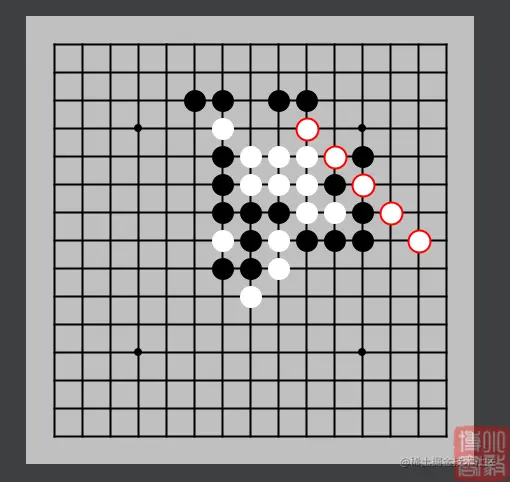• 活三又活二
• 冲四又活二
• 两个或两个以上的活三
• 冲四又活三

• 活三又活二：总分x2
• 冲四又活二：总分x4
• 两个或两个以上的活三：总分x6
• 冲四又活三：总分x8

``````    /**
* 检查当前局势是否处于某个局势
*
* @param situation  当前局势
* @param chessModel 检查的局势
* @return
*/private boolean checkSituation(String situation, ChessModel chessModel) {for (String value : chessModel.values) {if (situation.contains(value)) {return true;
}
}return false;
}``````

``````    /**
* 对当前棋位进行评估
*
* @param point 当前棋位
* @return
*/private int evaluate(Point point) {// 分值int score = 0;// 活三数int huosanTotal = 0;// 冲四数int chongsiTotal = 0;// 活二数int huoerTotal = 0;for (int i = 1; i < 5; i++) {
String situation = getSituation(point, i);if (checkSituation(situation, ChessModel.HUOSAN)) {// 活三+1
huosanTotal++;
} else if (checkSituation(situation, ChessModel.CHONGSI)) {// 冲四+1
chongsiTotal++;
} else if (checkSituation(situation, ChessModel.HUOER)) {// 活二+1
huoerTotal++;
}// 下此步的得分
score += getScore(situation);
}if (huosanTotal > 0 && huoerTotal > 0) {// 活三又活二
score *= 2;
}if (chongsiTotal > 0 && huoerTotal > 0) {// 冲四又活二
score *= 4;
}if (huosanTotal > 1) {// 活三数大于1
score *= 6;
}if (chongsiTotal > 0 && huosanTotal > 0) {// 冲四又活三
score *= 8;
}return score;
}``````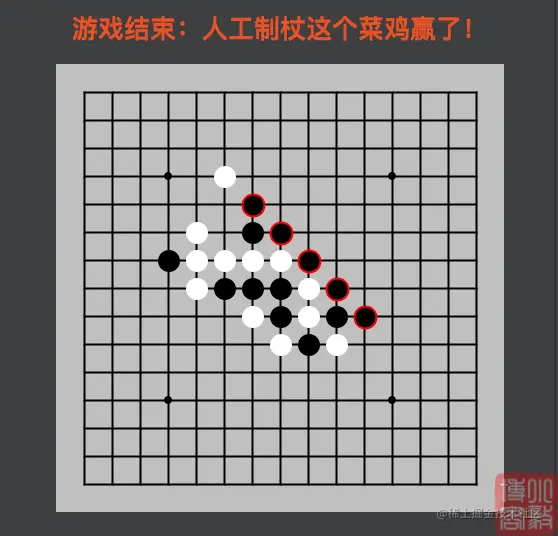`AI` 执黑的情况，赢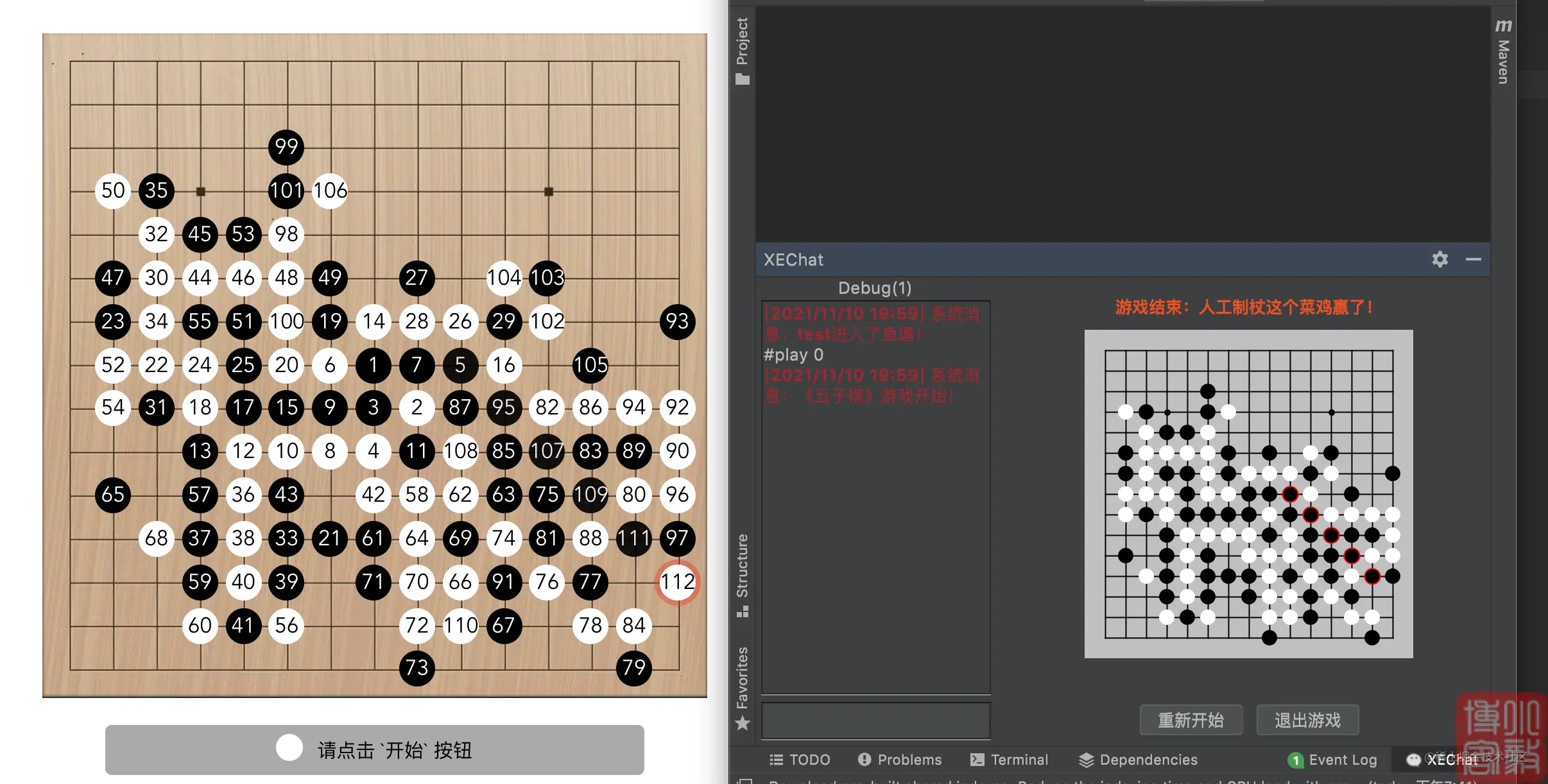`AI` 执白的情况，输